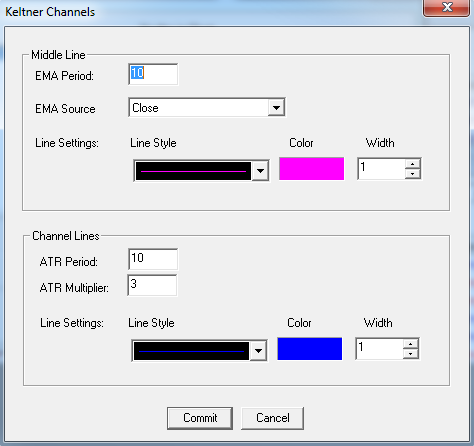# Keltner Channels

User can add Keltner Channels indicator to their chart by right click the chart and select Study Config to open the study configure window first, then select the “Keltner Channels” from the study list and add it into the Studies In Chart field.Keltner Channels Configure window allows user to modify the Keltner Channels chart, such as change the study period and lines’ color. User can open this Keltner Channels Configure window either by click the “Config” button or double click the study “Keltner Channels” under Studies In Chart section.

## Explanation of Each Field

·         Middle Line section allows user to adjust the middle line’s study parameters in Keltner Channels study.

Ø  EMA Period: Enter the study period for exponential moving average.

Ø  EMA Source: Use the dropdown menu to select the study source for the exponential moving average.

§  Open: Use the open price data to calculate the exponential moving average.

§  High: Use the highest price data to calculate the exponential moving average.

§  Low: Use the lowest price data to calculate the exponential moving average.

§  Close: Use the close price data to calculate the exponential moving average.

§  (High+Low)/2: Use this equation to calculate the price first, then use those price data to calculate the exponential moving average.

§  (High+Low+Close)/3: Use this equation to calculate the price first, then use those price data to calculate the exponential moving average.

§  (Open+High+Low+Close)/4: Use this equation to calculate the price first, then use those price data to calculate the exponential moving average.

Ø  Line Settings: Allows user to modify the middle line’s line style, line color, and line width.

·         Channel Lines section allows user to adjust the channel lines’ study parameters in Keltner Channels study.

Ø  ATR Period: Allows user to adjust the Average True Range (ATR)’s study period for channel lines in Keltner Channels study.

Ø  ATR Multiplier: Allows user to adjust the Average True Range (ATR)’s study multiplier for channel lines in Keltner Channels study.

Ø  Line Settings: allows user to modify the channel lines’ line style, line color, and line width.